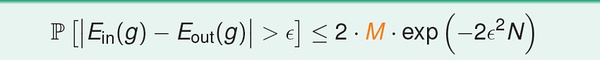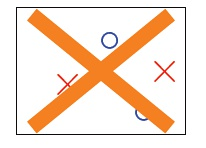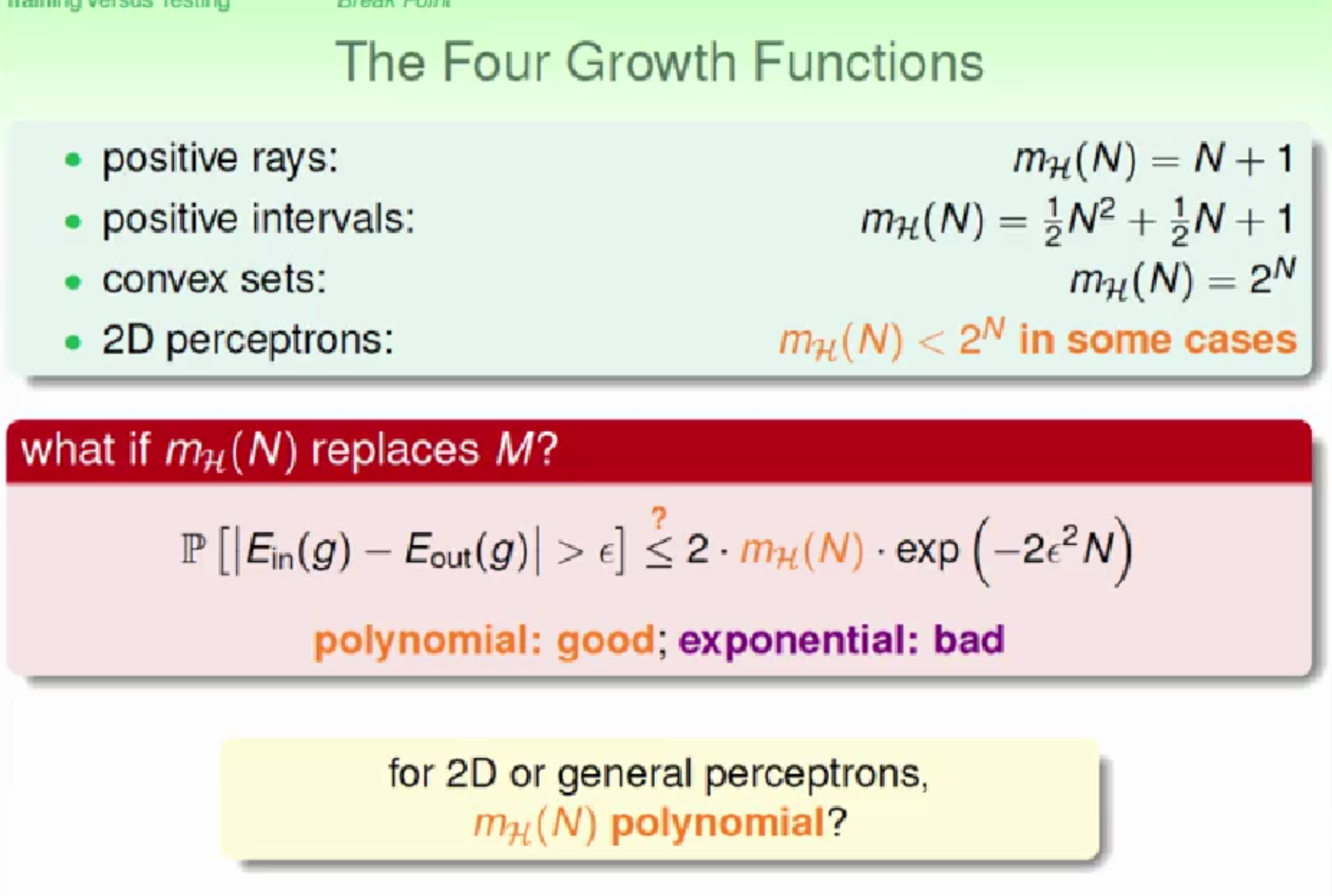# 机器学习基石之训练与测试

Posted by jjx on February 11, 2017（1） 假设空间H有限（M），且训练数据足够大，则可以保证测试错误率Eout 约等于训练错误率Ein；
（2）如果能得到Ein 接近于零，根据（1），Eout 趋向于零。

（1）当M 很小，那么坏数据出现的概率非常小（见第四讲分析），学习是有效的；但是由于假设空间过小，我们不一定能找到一个方案，可以使训练误差接近零；

（2）反之，若M 很大，坏数据出现的概率会变大。

（1）最简单的情形，只有一个输入数据时，我们最多只有两种假设：h1(x) = +1 or h2(x) = -1 。
（2）输入数据增加到两个，最多可以有四种假设：
h1(x1)=1, h1(x2)=1;
h2(x1)=-1, h2(x2)=1;
h3(x1)=1, h3(x2)=-1;
h4(x1)=-1, h4(x2)=-1.（圆圈和叉代表两种不同分类，这种情形也就是线性不可分）。

（1）X=R（一维实数空间），h(x) = sign(x-a)， a 是参数。

（2）X=R， h(x) = 1 iff x>=a or x<b, -1 otherwise. 有两个参数 a, b.

（3）X=R^2（二维实数空间），h是一个任意凸多边形，多边形内部的为1，外部的为-1。